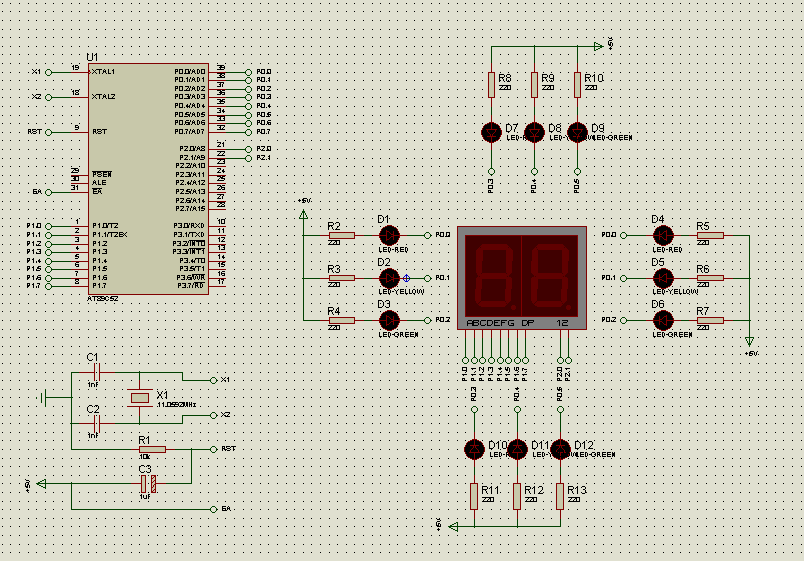51单片机交通灯的proteus仿真怎么弄

#include
#define uchar unsigned char
#define uint unsigned int
sbit dula=P2^6;
sbit wela=P2^7;
sbit dx_hong=P0^0;
sbit dx_huang=P0^1;
sbit dx_lv=P0^2;
sbit nb_hong=P0^3;
sbit nb_huang=P0^4;
sbit nb_lv=P0^5;
uchar a,shi,ge,num1,num2,num3,num4,num5,num6;
uchar code table[]={
0x3f,0x06,0x5b,0x4f,
0x66,0x6d,0x7d,0x07,
0x7f,0x6f,0x77,0x7c,
0x39,0x5e,0x79,0x71};
void delayms(uint xms)
{
uint i,j;
for(i=xms;i>0;i--)
for(j=110;j>0;j--);
}
void display(uchar shi,uchar ge)
{
wela=1;
P2=0xfe;
wela=0;
P1=0xff;
dula=1;
P1=table[shi];
dula=0;
delayms(5);

``````wela=1;
P2=0xfd;
wela=0;
P1=0xff;
dula=1;
P1=table[ge];
dula=0;
delayms(5);
``````

}
void djs(uchar xs)
{
if(num2>xs)
{
num2=0;
}
shi=(xs-num2)/10;
ge=(xs-num2)%10;
display(shi,ge);
}
void init()
{
TMOD=0x11;
TH0=(65536-45872)/256;
TL0=(65536-45872)%256;
EA=1;
ET0=1;
TR0=1;

}
void main()
{

``````while(1)
{
init();

dx_hong=0;
dx_huang=1;
dx_lv=1;
nb_hong=1;
nb_huang=1;
nb_lv=0;
djs(60);

dx_hong=1;
dx_huang=0;
dx_lv=1;
nb_hong=1;
nb_huang=0;
nb_lv=1;
djs(30);

dx_hong=1;
dx_huang=1;
dx_lv=0;
nb_hong=0;
nb_huang=1;
nb_lv=1;
djs(60);

dx_hong=1;
dx_huang=0;
dx_lv=1;
nb_hong=1;
nb_huang=0;
nb_lv=1;
djs(30);
}
``````

}
void T0_time()interrupt 1
{
TH0=(65536-45872)/256;
TL0=(65536-45872)%256;
num1++;
if(num1==20)
{
num1=0;
num2++;

}
}0

1个回答

051单片机 的交通灯仿真与原理图
51单片机 交通灯 仿真与原理图 51单片机 交通灯 仿真与原理图51单片机 交通灯 仿真与原理图

protues仿真51单片机，带源码，电路图，有中文注释----模拟交通灯

51单片机交通灯 源代码+proteus仿真图

//////////////////////////////////////////////////////////////////////////// //                       蝙蝠龙51单片机开发学习系统                       // ////////////////////////////////////////////////////////

mcs 51单片机 proteus 仿真实用实例大全 例子集合，含汇编代码keil工程和proteus工程

8086交通灯_基于Proteus仿真

51单片机Protues，仿真交通灯。程序+仿真
51单片机Protues，仿真交通灯。程序+仿真

Keil和Proteus的交通灯仿真实例（提供程序源码）

51单片机模拟交通灯

51单片机超级交通灯proteus仿真
51单片机超级交通灯proteus仿真
51 汇编编程：倒计时交通灯

51单片机设计交通灯（仿真+C程序
51单片机的交通灯设计，通过C仿真和protues实现

51单片机完整开发板的proteus仿真，供大家学习参考

51单片机230个Proteus仿真实例源码下载
51单片机230个Proteus仿真实例

51单片机的交通灯protues仿真原理图